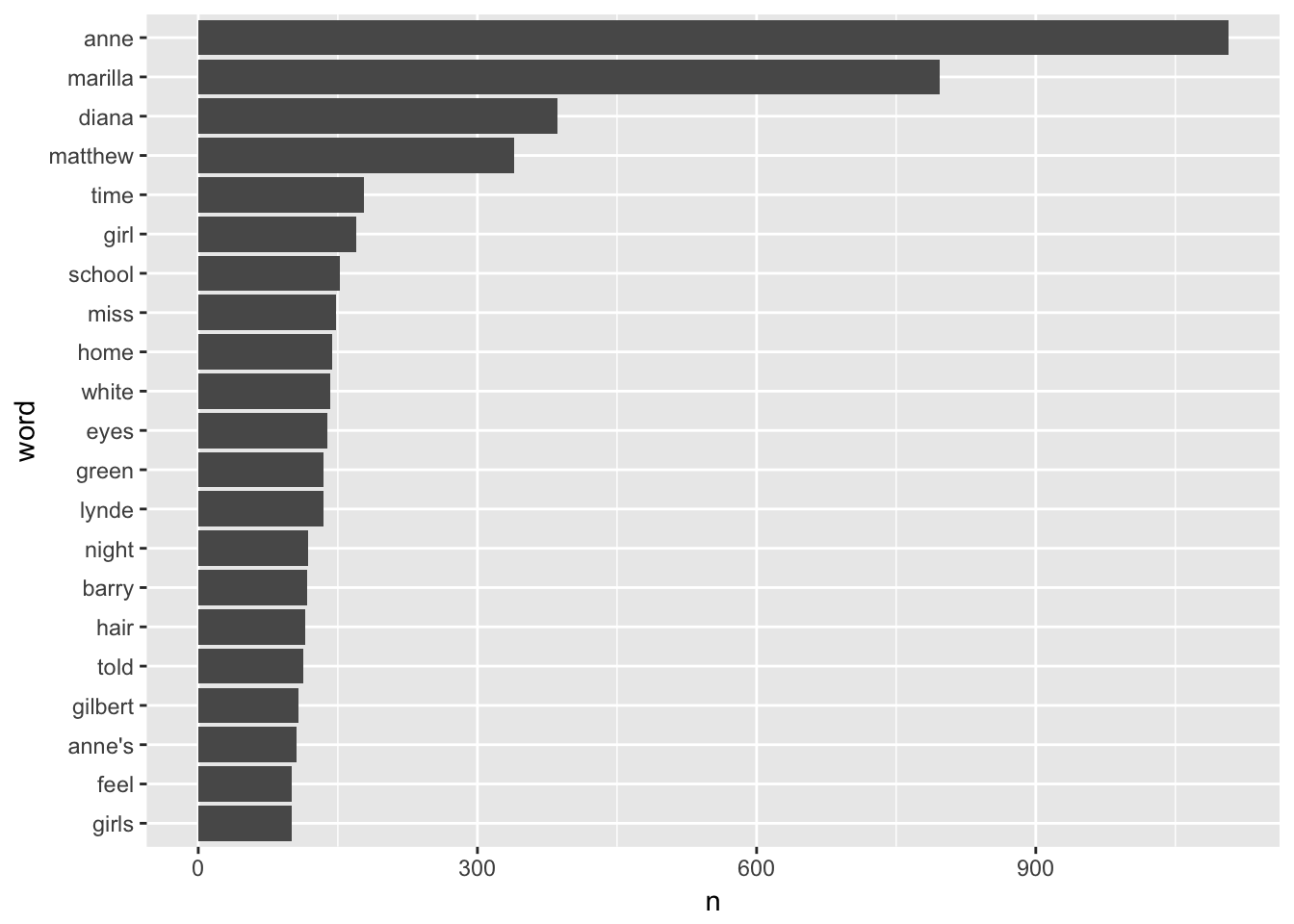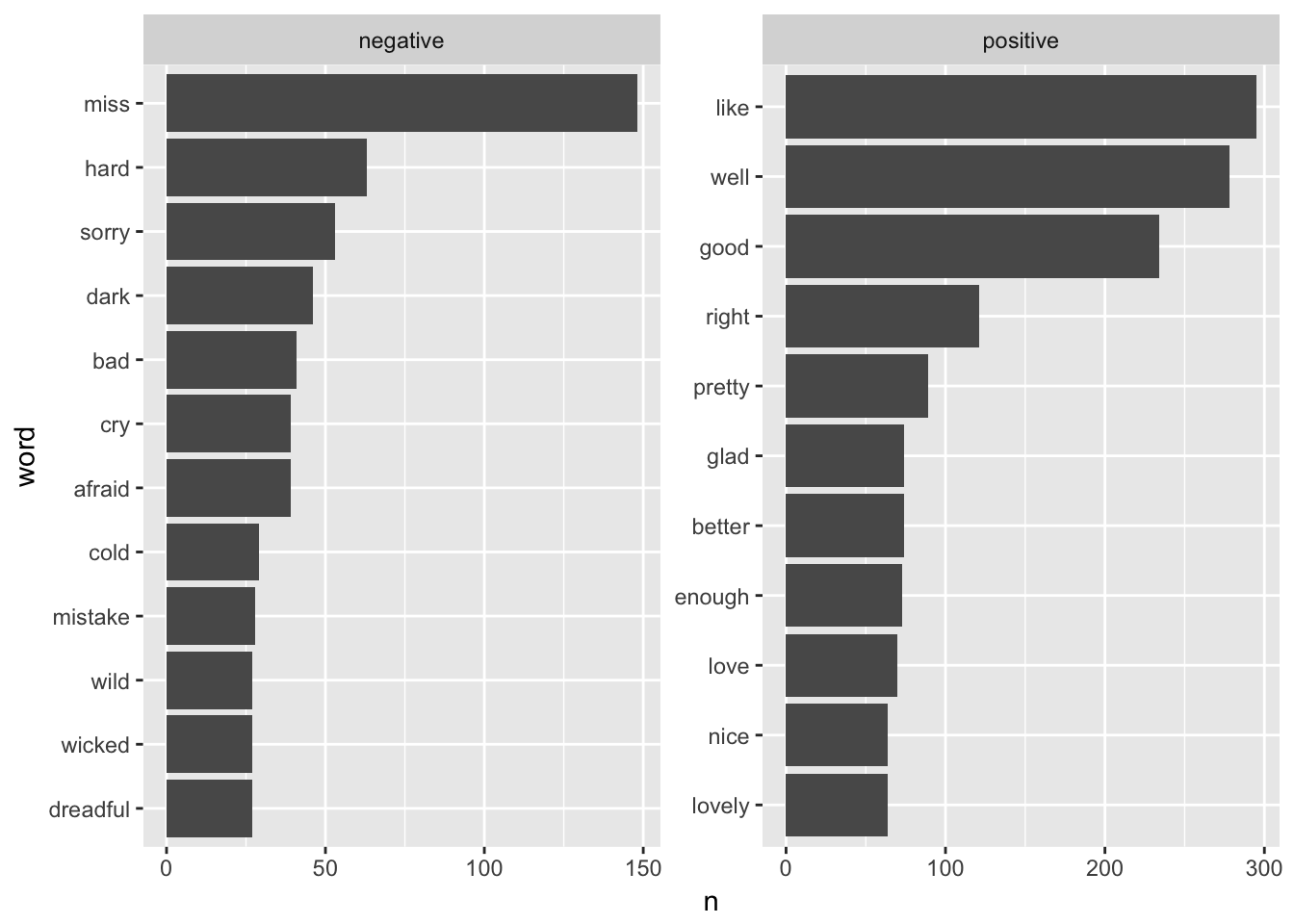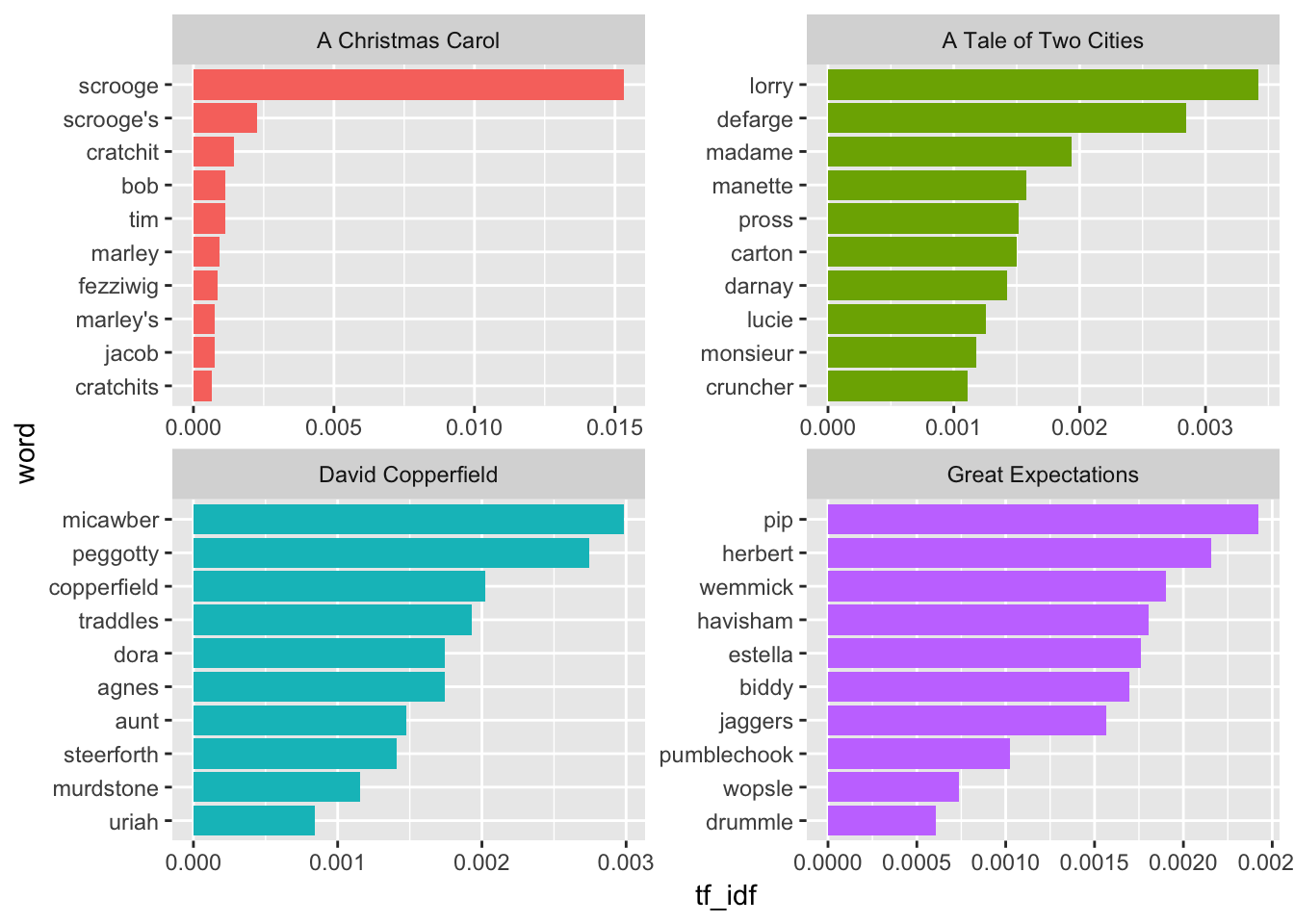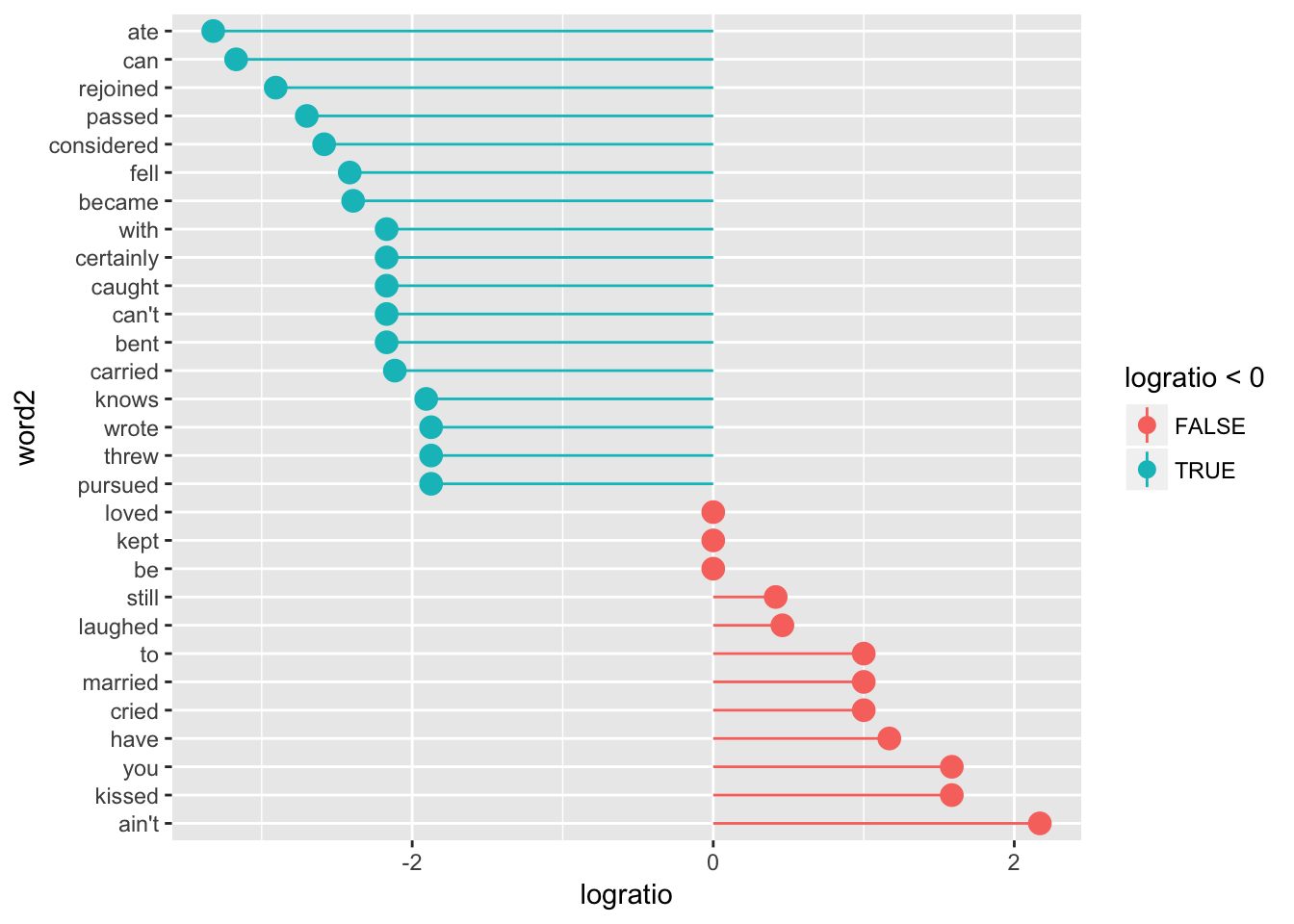# Surveys and qualitative data

Materials for class on Tuesday, October 24, 2017

## Text analysis and visualization

``````library(tidyverse)
library(tidytext)
library(gutenbergr)
library(forcats)``````

We can download any book from Project Gutenberg with `gutenbergr::gutenberg_download()`. The `gutenberg_id` argument is the ID for the book, found in the URL for the book. In class we looked at Anne of Green Gables, which has an ID of 45.

``book <- gutenberg_download(45)``

Once we’ve downloaded the book, we can tokenize the text (i.e. divide into words), and then create a long tidy data frame. `tidytext` does simple tokenization—it will not determine parts of speech or anything fancy like that. Look at the `cleanNLP` package for a tidy way to get full-blown natural language processing into R.

``````tidy_book <- book %>%
mutate(line = row_number()) %>%
unnest_tokens(word, text)

tidy_book %>% head() %>% knitr::kable()``````
gutenberg_id line word
45 1 anne
45 1 of
45 1 green
45 1 gables
45 3 by
45 3 lucy

### Word frequencies

We can filter out common stopwords and then view the 20 most frequent words:

``````plot_words <- tidy_book %>%
anti_join(stop_words, by = "word") %>%
count(word, sort = TRUE) %>%
top_n(20, n) %>%
mutate(word = fct_rev(fct_inorder(word, ordered = TRUE)))

ggplot(plot_words, aes(x = word, y = n)) +
geom_col() +
coord_flip()``````### Sentiment analysis

There are several existing dictionaries of word sentiments, such as AFINN and Bing, which work differently—some use a continuous scale of negativity-positivity, whil others use a dichotomous variable:

``get_sentiments("afinn") %>% head() %>% knitr::kable()``
word score
abandon -2
abandoned -2
abandons -2
abducted -2
abduction -2
abductions -2
``get_sentiments("bing") %>% head() %>% knitr::kable()``
word sentiment
2-faced negative
2-faces negative
a+ positive
abnormal negative
abolish negative
abominable negative

We can join one of these sentiment dictionaries to the list of words and find the most common positive and negative words:

``````plot_sentiment <- tidy_book %>%
inner_join(get_sentiments("bing"), by = "word") %>%
count(sentiment, word, sort = TRUE) %>%
group_by(sentiment) %>%
top_n(10, n) %>%
ungroup() %>%
arrange(sentiment, n) %>%
mutate(word = fct_inorder(word))

ggplot(plot_sentiment, aes(x = word, y = n)) +
geom_col() +
coord_flip() +
facet_wrap(~ sentiment, scales = "free")``````### tf-idf: term frequency—inverse document frequency

Calculating the tf-idf lets us find the most unique words in individual documents in a collection, relative to other documents in the collection. Here we download four Dickens novels (A Tale of Two Cities (98), David Copperfield (766), Great Expectations (1400), and A Christmas Carol (19337)) and combine them into a tidy corpus:

``````dickens <- gutenberg_download(c(19337, 98, 1400, 766),
meta_fields = "title")``````

We can then calculate the tf-idf across the different books:

``````dickens_tidy <- dickens %>%
unnest_tokens(word, text) %>%
count(title, word, sort = TRUE)

dickens_unique <- dickens_tidy %>%
bind_tf_idf(word, title, n)

unique_top_10 <- dickens_unique %>%
group_by(title) %>%
top_n(10, tf_idf) %>%
ungroup() %>%
arrange(title, tf_idf) %>%
mutate(word = fct_inorder(word))

unique_top_10 %>% head() %>% knitr::kable()``````
title word n tf idf tf_idf
A Christmas Carol cratchits 14 0.0004730 1.386294 0.0006557
A Christmas Carol jacob 16 0.0005406 1.386294 0.0007494
A Christmas Carol marley’s 16 0.0005406 1.386294 0.0007494
A Christmas Carol fezziwig 18 0.0006081 1.386294 0.0008430
A Christmas Carol marley 20 0.0006757 1.386294 0.0009367
A Christmas Carol tim 24 0.0008108 1.386294 0.0011241
``````ggplot(unique_top_10, aes(x = word, y = tf_idf, fill = title)) +
geom_col(show.legend = FALSE) +
coord_flip() +
facet_wrap(~ title, scales = "free")``````### n-grams

We can also find the most common pairs of words, or n-grams. Rather than tokenizing by word, we can tokenize by ngram and specify the number of words—here we want bigrams, so we specify `n = 2`.

``````dickens_bigrams <- dickens %>%
unnest_tokens(bigram, text, token = "ngrams", n = 2) %>%
count(bigram, sort = TRUE)

dickens_bigrams %>% head() %>% knitr::kable()``````
bigram n
of the 3261
in the 3226
it was 1756
to be 1643
that i 1641
to the 1633

In class, we were interested in seeing which words are more likely to appear after “he” and “she” to see if there are any gendered patterns in Dickens’ novels (similar to this and this). To do this, we separate the bigram column into two columns named `word1` and `word2`, and filter the data so that it only includes rows where `word1` is “he” or “she”.

We then calculate the log odds for each pair of words to see which ones are more likely to appear across genders. We finally sort the data by the absolute value of the log ratio (since some are negative) and take the top 15.

``````dickens_bigrams <- dickens_bigrams %>%
separate(bigram, c("word1", "word2"), sep = " ") %>%
filter(word1 %in% c("he", "she")) %>%
count(word1, word2, wt = n, sort = TRUE) %>%
rename(total = nn)

dickens_ratios <- dickens_bigrams %>%
group_by(word2) %>%
filter(sum(total) > 10) %>%
ungroup() %>%
spread(word1, total, fill = 0) %>%
mutate(logratio = log2(she / he)) %>%
arrange(desc(logratio))

plot_ratios <- dickens_ratios %>%
mutate(abslogratio = abs(logratio)) %>%
group_by(logratio < 0) %>%
top_n(15, abslogratio) %>%
ungroup() %>%
arrange(desc(logratio)) %>%
mutate(word2 = fct_inorder(word2, ordered = TRUE))

ggplot(plot_ratios, aes(x = word2, y = logratio, color = logratio < 0)) +
geom_pointrange(aes(ymin = 0, ymax = logratio), size = 0.5, fatten = 6) +
coord_flip()``````## Feedback for today

First, make sure you fill out BYU’s official ratings for this class sometime before Saturday, October 28.

Second, go to this form and answer these questions (anonymously if you want):

1. What were the two most important things you learned in this class?
2. What were the two most exciting things you learned in this class?
3. What were the two most difficult things you had to do in this class?
4. Which class sessions were most helpful? Which were least helpful?
5. Which readings were most helpful? Which were least helpful?
6. What should I remove from future versions of this class?
7. What should I add to future versions of this class?
8. What else should I change in future versions of this class?
9. Any other comments?